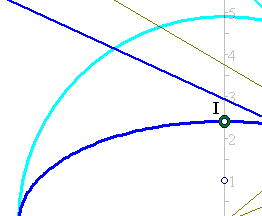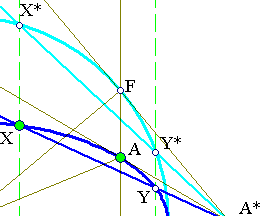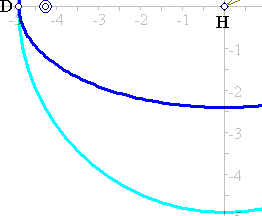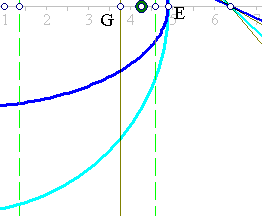##Common Polar

Given the ellipse with axes a=HE, b=HI (a>b) and equation x˛/a˛ + y˛/b˛ =1. The A* on the x-axis (line of major axis of the ellipse) have the same polar with respect to the ellipse and with respect to the auxiliary circle of the ellipse (see Auxiliary.html for this circle).In fact, consider a point A on the ellipse and the corresponding point F on the auxiliary circle, having the same ordinate x with A. The tangents of the ellipse at A and the auxiliary circle at F respectively intersect at the same point A* of the major axis. This is discussed in Ellipse.html . This implies easily that the polars of points A* on the x-axis are the same with respect to both the ellipse and its auxiliary circle. This has an interesting consequence. Consider two points X, Y of the ellipse defining a chord, which passes through the point A* of the x-axis. Consider also the corresponding points X*, Y* of the auxiliary circle, having the same abscissas with these points. Then the chord of the circle through X*, Y* passes also trhough A*.
For the relation of the polars for points A* other than the points of x-axis see the file CommonPolar2.html .